# Texas Go Math Grade 4 Lesson 15.3 Answer Key Customary Units of Weight

Refer to our Texas Go Math Grade 4 Answer Key Pdf to score good marks in the exams. Test yourself by practicing the problems from Texas Go Math Grade 4 Lesson 15.3 Answer Key Customary Units of Weight.

## Texas Go Math Grade 4 Lesson 15.3 Answer Key Customary Units of Weight

Essential Question

How can you convert customary units of weight?
In the customary system of measurement, the most common units of weight are the ounce (oz) and pound (lb)

Unlock the Problem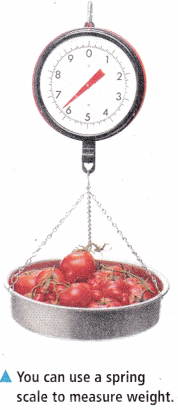Ounces and pounds are customary units of weight. How does the size of a pound compare to the size of an ounce?
1 pound = 16 ounces

Activity

Materials

• color pencils

The number line below shows the relationship between pounds and ounces.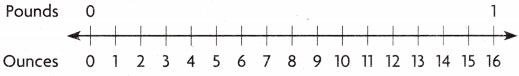STEP 1 Use a color pencil to shade 1 pound on the number line.

STEP 2 Use a different color pencil to shade 1 ounce on the number line.

STEP 3 Compare the size of 1 pound to the size of 1 ounce.
You need _________ ounces to make _________ pound.
So, 1 pound is _________ times as heavy as 1 ounce.
Explanation:
You need 16 ounces to make 1 pound.
So, 1 pound is 16 times as heavy as 1 ounce.

Explain how the number line helped you to compare the sizes of the units.Explanation:
With the help of number line we can easily understand
1 pound = 16 ounces

Math Talk

Mathematical Processes
Which is greater, 9 pounds or 9 ounces? Explain.
9 pounds is great
Explanation:
1 pound = 16 ounces

Example 1 Convert larger units to smaller units.

Nancy needs 5 pounds of flour to bake pies for a festival.
How many ounces of flour does Nancy need to bake the pies?

STEP 1 Make a table that relates pounds and ounces.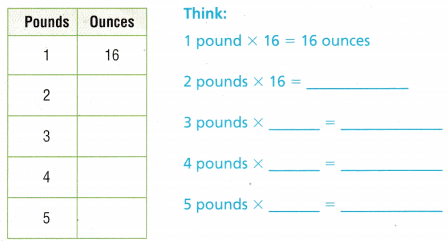So, Nancy needs _________ ounces of flour.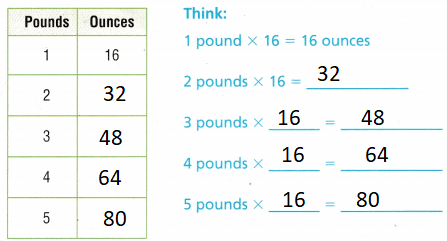Explanation:
So, Nancy needs 80 ounces of flour.

Example 2 Convert smaller units to larger units.

Marcel buys 144 ounces of sugar at the grocery store. How many pounds of sugar did Marcel buy?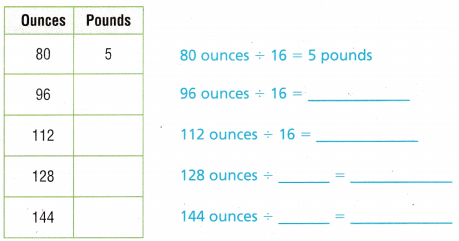So, Marcel bought ____________ pounds of sugar.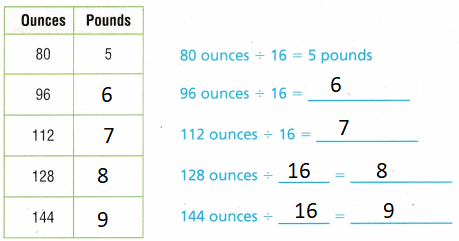Explanation:
So, Marcel bought 9 pounds of sugar.

Try This! There are 2,000 pounds in 1 ton.
Make a table that relates tons and pounds.1 ton is ____________ times as heavy as 1 pound.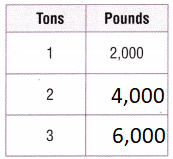Explanation:
1 ton is 2000 times as heavy as 1 pound.

Share and ShowQuestion 1.
4 tons = _________ pounds
Think: 4 tons × _________ = _________
4 tons = 8000 pounds
Explanation:
1 ton= 2000 pounds
4 x 2000 = 8000 pounds

Complete.

Question 2.
5 tons = _________ pounds
Explanation:
1 ton= 2000 pounds
5 x 2000 = 10000 pounds

Go Math Answer Key Grade 4 Customary Units of Weight Question 3.
6 pounds = _________ ounces
Explanation:
1 pound= 16 ounces
6 x 16= 96 ounces

Question 4.
48 ounces = _________ pounds
Explanation:
1 pound= 16 ounces
48 ÷ 16 = 3 pounds

Question 5.
128 ounces = _________ pounds
Explanation:
1 pound= 16 ounces
128 ÷ 16 = 8 pounds

Math Talk

Mathematical Processes
What equation can you use to solve Exercise 3? Explain.
A multiplication equation is used
for exercise 3
6 x 16= 96 ounces

Complete.

Question 6.
A landscaping company ordered 8 tons of gravel. They sell the gravel in 50-pound bags. How many pounds of gravel did the company order?
They ordered 16000 pounds
Explanation:
8 x 2000 = 16000
16000 ÷ 50 = 320
They sell 320 bags of 50 pounds

Question 7.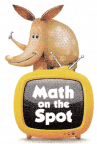H.O.T. Communicate If you could draw a number line that shows the relationship between tons and pounds, what would it look like? Explain.Explanation:
1 pound = 16 ounces
The number line is drawn to show the relationship between pounds and ounces.

H.O.T. Multi-Step Keith bought 4 steaks from the store. One steak was 14 ounces, one steak was 12 ounces, one steak was 8 ounces and the last steak was 14 ounces. How many pounds of steak did Keith buy from the grocery store? Explain.
Explanation:
total steaks weight = 14 +12 + 8 + 14 = 48 ounces
1 pound= 16 ounces
48 ÷ 16 = 3

Question 9.
Jill needs 4 pounds of peanut butter to make peanut butter and jelly sandwiches for a party. She buys peanut butter in jars. The weight of peanut butter in jars is given in ounces. How many ounces of peanut butter does Jill need to make the sandwiches?
(A) 4 ounces
(B) 16 ounces
(C) 8 ounces
(D) 64 ounces
Explanation:
1 pound= 16 ounces
4  x 16=  64 ounces

Question 10.
The vet tells Jan that her puppy weighs 5 pounds. The scale shows the puppy’s weight in ounces. What weight does the scale show?
(A) 5 ounces
(B) 80 ounces
(C) 16 ounces
(D) 60 ounces
Explanation:
1 pound= 16 ounces
5 x 16=  80 ounces

Question 11.
Multi-Step A truck is transporting 4 new cars to a car dealership. Each car weighs 2 tons. The weight limit for trucks going over bridges is given in pounds. What is the weight of the cars in pounds?
(A) 16 pounds
(B) 8,000 pounds
(C) 16,000 pounds
(D) 24,000 pounds
Explanation:
1 ton= 2000 pounds
4 cars x 2 tons = 8 tons
8 x 2000 = 16000 pounds

TEXAS Test Prep

Question 12.
Mike is recording his baby sister’s weight in pounds and ounces each week. This week she weighs 10 pounds. How many ounces does she weigh?
(A) 160 ounces
(B) 10 ounces
(C) 20 ounces
(D) 16 ounces
Explanation:
1 pound= 16 ounces
10 x 16= 160 ounces

### Texas Go Math Grade 4 Lesson 15.3 Homework and Practice Answer Key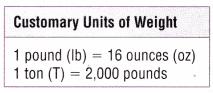Complete.

Question 1.
48 ounces = _____________ pounds
Explanation:
1 pound= 16 ounces
48 ÷ 16 = 3 pounds

Question 2.
3 tons = _____________ pounds
Explanation:
1 ton= 2000 pounds
3 x 2000 = 6000 pounds

Question 3.
7 pounds = _____________ ounces
Explanation:
1 pound= 16 ounces
7 x 16= 112 ounces

Question 4.
10,000 pounds = _____________ tons
Explanation:
1 ton= 2000 pounds
10000 ÷2000 = 5 tons

Grade 4 Lesson 15.3 Go Math Customary Units of Weight Question 5.
7 tons = _____________ pounds
Explanation:
1 ton= 2000 pounds
7 x 2000 = 14000 pounds

Question 6.
96 ounces = _____________ pounds
Explanation:
1 pound= 16 ounces
96 ÷ 16 =6 pounds

Question 7.
64 ounces = _____________ pounds
Explanation:
1 pound= 16 ounces
64 ÷ 16 =4 pounds

Question 8.
8pounds = _____________ ounces
Explanation:
1 pound= 16 ounces
16 x 8=128

Question 9.
Nathan has a truck that can carry 2 tons. How many pounds can the truck carry?
Explanation:
1 ton= 2000 pounds
2 x 2000 = 4000 pounds

Question 10.
Julia bought 40 bags of compost. Each bag weighed 50 pounds. How many tons of compost did she buy?
Explanation:
40 bags x 50 pounds = 2000 pounds
1 ton= 2000 pounds

Question 11.
Paul bought 3 packages of cheese. The packages weighed 11 ounces, 13 ounces, and 8 ounces. How many pounds of cheese did Paul buy? Explain.
Explanation:
Total weight is 11+13+8 = 32 ounces
1 pound= 16 ounces
32 ÷ 16 =2

Question 12.
Carole needs 4 pounds of nuts for her granola. She has 26 ounces of walnuts and 28 ounces of cashews. How many ounces of peanuts. should she buy so she has 4 pounds of nuts? Explain.
Explanation:
1 pound= 16 ounces, So Total she needs
4 x 16 = 64 ounces of nuts.
She has  26 ounces of walnuts and 28 ounces of cashews. So total she has
26  + 28 = 54 ounces
She needs to buy more 10 ounces since
64 -54 =10

Lesson Check

Fill in the bubble completely to show your

Question 13.
How many ounces of potatoes are in a 20 pound bag of potatoes?
(A) 40,000 ounces
(B) 3,200 ounces
(C) 320 ounces
(D) 2,000 ounces
Explanation:
1 pound= 16 ounces
16 x  20=3200

TEXAS Test Prep

Question 14.
A construction company orders 12 tons of pea gravel. How many pounds of pea gravel do they order?
(A) 6,000 pounds
(B) 24,000 pounds
(C) 192 pounds
(D) 32,000 pounds
Explanation:
1 ton= 2000 pounds
12 x 2000 = 24000 pounds

Question 15.
At the farmer’s market, a bunch of bananas weighs 96 ounces. The price for the bananas is based on the weight in pounds. How many pounds does the bunch of bananas weigh?
(A) 6 pounds
(§) 12 pounds
(C) 1,536 pounds
(D) 96 pounds
Explanation:
1 pound= 16 ounces
96 ÷ 16 = 6 pounds

Which statement about 36 ounces and 2 pounds is true?
(A) 36 ounces > 2 pounds
(B) 2 pounds = 36 ounces
(C) 36 ounces < 2 pounds
(D) 2 pounds > 36 ounces
Explanation:
1 pound= 16 ounces
2 pounds x 16 ounces = 32 ounces
36 ounces greater than 32 ounces so option A is the answer.

Question 17.
Multi-Step Elizabeth has 4 jars of olives. Each jar contains 12 ounces of olives. How many pounds of olives does Elizabeth have?
(A) 48 pounds
(B) 4 pounds
(C) 3 pounds
(D) 12 pounds
Explanation:
1 pound= 16 ounces
4 jars x 12 ounces = 48 ounces
48 ÷ 16 = 3
Question 18.
Multi-Step Kevin picked 10 pounds of strawberries. He wants to freeze them in 8-ounce packages. How many 8-ounce packages of strawberries can Kevin make?
(A) 16
(B) 32
(C) 80
(D) 20